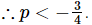Find all the values of p, such that 6 lies s...
Find all the values of p, such that 6 lies some where between the roots of the equation
x2 + 2(p - 3)x + 9 = 0. (‘x’ is a real number).
• a)
p < />
• b)
P > 6
• c)
0 < p="" />< />
• d)
0 < p="" />< />
Find all the values of p, such that 6 lies some where between the roo...
Both the roots will be real as 6 lies between the roots
(i) b2 − 4ac > 0
= 4(p − 3)2 − 4 × 9 > 0P(P − 6) > 0
p > 6 or p < />
(ii), since x = 6 lies between the two roots.
so, (6)2 + 2(p − 3)6 + 9 < />Hence the correct option is A.
 1 Crore+ students have signed up on EduRev. Have you?
1 Crore+ students have signed up on EduRev. Have you?

### Learn this topic in detailIPMAT Mock Test - 8 (New Pattern) 100 Ques | 90 MinsMore from Related Course Quantitative Aptitude (Quant)View courses related to this question

### Quick links for CAT exam850+
Video Lectures2500+
Revision Notes600+
Online Tests10,000+
Doubts Solved
Find all the values of p, such that 6 lies some where between the roots of the equationx2 + 2(p - 3)x + 9 = 0. (‘x’ is a real number).a)p b)P > 6c)0 d)0 Correct answer is option 'A'. Can you explain this answer?
Question Description
Find all the values of p, such that 6 lies some where between the roots of the equationx2 + 2(p - 3)x + 9 = 0. (‘x’ is a real number).a)p b)P > 6c)0 d)0 Correct answer is option 'A'. Can you explain this answer? for CAT 2023 is part of CAT preparation. The Question and answers have been prepared according to the CAT exam syllabus. Information about Find all the values of p, such that 6 lies some where between the roots of the equationx2 + 2(p - 3)x + 9 = 0. (‘x’ is a real number).a)p b)P > 6c)0 d)0 Correct answer is option 'A'. Can you explain this answer? covers all topics & solutions for CAT 2023 Exam. Find important definitions, questions, meanings, examples, exercises and tests below for Find all the values of p, such that 6 lies some where between the roots of the equationx2 + 2(p - 3)x + 9 = 0. (‘x’ is a real number).a)p b)P > 6c)0 d)0 Correct answer is option 'A'. Can you explain this answer?.
Solutions for Find all the values of p, such that 6 lies some where between the roots of the equationx2 + 2(p - 3)x + 9 = 0. (‘x’ is a real number).a)p b)P > 6c)0 d)0 Correct answer is option 'A'. Can you explain this answer? in English & in Hindi are available as part of our courses for CAT. Download more important topics, notes, lectures and mock test series for CAT Exam by signing up for free.
Here you can find the meaning of Find all the values of p, such that 6 lies some where between the roots of the equationx2 + 2(p - 3)x + 9 = 0. (‘x’ is a real number).a)p b)P > 6c)0 d)0 Correct answer is option 'A'. Can you explain this answer? defined & explained in the simplest way possible. Besides giving the explanation of Find all the values of p, such that 6 lies some where between the roots of the equationx2 + 2(p - 3)x + 9 = 0. (‘x’ is a real number).a)p b)P > 6c)0 d)0 Correct answer is option 'A'. Can you explain this answer?, a detailed solution for Find all the values of p, such that 6 lies some where between the roots of the equationx2 + 2(p - 3)x + 9 = 0. (‘x’ is a real number).a)p b)P > 6c)0 d)0 Correct answer is option 'A'. Can you explain this answer? has been provided alongside types of Find all the values of p, such that 6 lies some where between the roots of the equationx2 + 2(p - 3)x + 9 = 0. (‘x’ is a real number).a)p b)P > 6c)0 d)0 Correct answer is option 'A'. Can you explain this answer? theory, EduRev gives you an ample number of questions to practice Find all the values of p, such that 6 lies some where between the roots of the equationx2 + 2(p - 3)x + 9 = 0. (‘x’ is a real number).a)p b)P > 6c)0 d)0 Correct answer is option 'A'. Can you explain this answer? tests, examples and also practice CAT tests.
Do you know? How Toppers prepare for CAT Exam
With help of the best CAT teachers & toppers, We have prepared a guide for student who are preparing for CAT : 15 Steps to clear CAT Exam(Scan QR code)